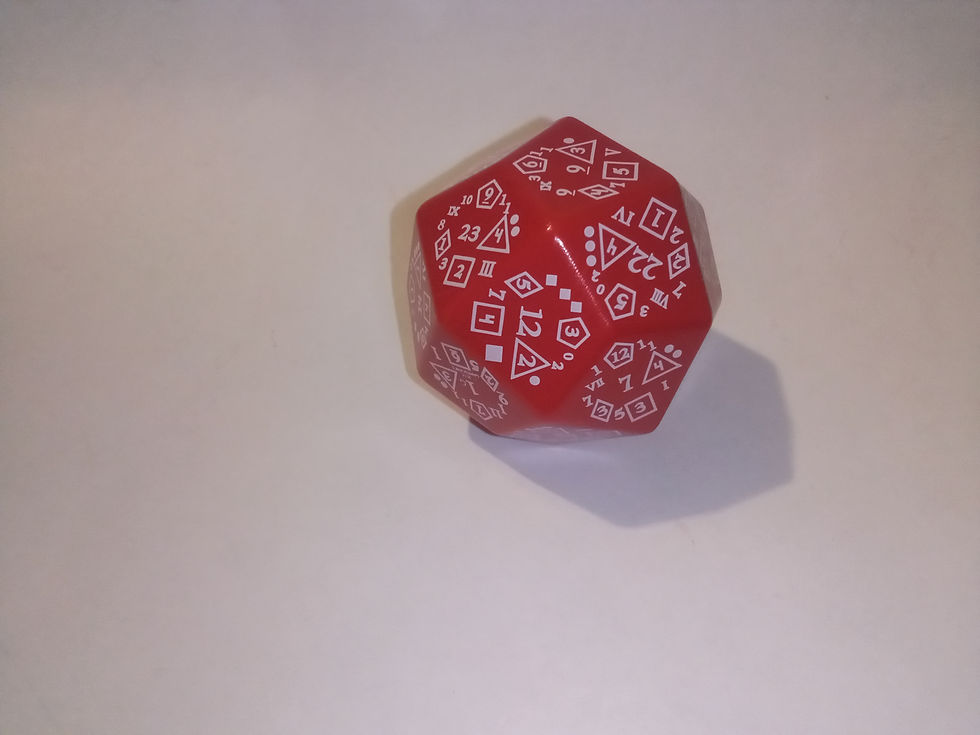top of page# Red D-Total (Modern).

SKU: D-T/GMS/Pla/Red
`Die you want to roll.How to read the face: die face is laid out like a clock face, so turn the dye so all numbers (on the face rolled) are right side up to you.D2: in the middle of the face, there is a number between 1 and 24. If 12:00 or less, the D2 has rolled a one. If 13 or higher, the result is a two.D3: the number of pips (spots) in the 3:00 position is the D3 result.D4: result lies in a triangle (traditional D4 shape) at 4:00 position.D5: result is a Roman numeral (I-V) at 5:00. If numeral is not there, reroll.D6: result is in a square (traditional d6 shape) at 6:00.D7: at 7:00 (bottom left) there may be a number (1-7) between the square in the diamond shapes. If not, reroll to get the D7 result.D8: result lies within a diamond (traditional d8 shape) at 8:00.D10: in the middle of the face there is a number (1-24). Roll until it lands on 1-20, and use the final digit for the d10 result. (A roll of five or 15 is considered a five; a roll of 10 or 20 is considered a 10)D12: result lies within pentagon (traditional D12 shape) at 12:00.*To read d20 and higher outcomes (except d24), reroll the dye if 21-24 is rolled.*D20: result is the number on the center of the face*D24: the middle number on the face is the d24 result.D30: uses D3 pips for first digit (one pip equals one through 10, 2 equals 11 through 20 and 3 equals 21 through 30). Reroll, using d10 method* for the second digit. First roll (D3 equals two pips) plus second roll (d10 equals 7 or 17) =17.This method is also used for the d40 through d80, except for the additional ranges of tens.D40: uses D4 triangle for first digit, with the method described above (but adding four equals 31 through 40). Reroll using d10 method* for second digit. [(D4 equals)+(d10 equals 16)=36]D50: uses D5 (Roman numerals) for first digit (V equals 41 through 50), and d10 method* for second digit (three or 13 equals 3). For example, a III and reroll of three equals 23.D60: uses d6 square for first digit and d10 method* for second digit. [(D4 equals 4) Plus (d10 equals 16) equals 36]D70: uses D7 position for first digit and d10 method* for the second. [(D7 equals) Plus (d10 equals 8) equals 68]D80: uses the d8 diamond for first digit (eight equals 71 through 80) and d10 method* for the second. For example, when d8 equals 8 in the d10 reroll equals 4 or 14, the result is 74.D100: if the first in the second roll is 10, you have rolled a 100. If the one or two is the first roll, you have rolled a tent or less number. Roll again. If 10 or 20 is rolled, you have a 10, otherwise use the single digit rolled. Use d10 method* twice, first for range of tins and second for final digit.`